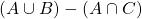# Mathematics - Discrete Mathematics - Set Operations

in STEMGeeks2 years ago[Image 1]

## Introduction

Hey it's a me again @drifter1!

Today we continue with Mathematics, and more specifically the branch of "Discrete Mathematics", in order to get into Set Operations.

I highly suggest checking out the previous part before this one...

So, without further ado, let's get straight into it!

## Venn Diagrams

Venn diagrams are illustrations which use circles for the purpose of representing the relationship between various things. If the things have common elements the circles will overlap.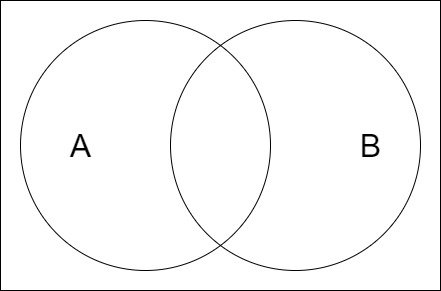Such diagrams are commonly used in set theory and logic theory to represent the relationship between sets and data, or the operations visually.

## Set Operations

Using Venn Diagrams, let's explain the basic operations on sets.

### Union

The union of sets A and B, denoted by A ∪ B, is a set which contains elements that belong to A or B or both. Visually: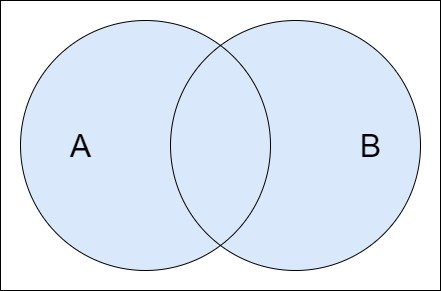### Intersection

The intersection of sets A and B, denoted by A ∩ B, is a set which contains elements that belong to both A and B. Visually: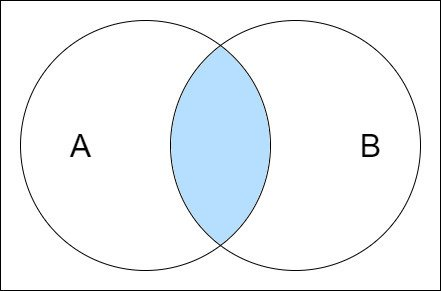### Difference

The difference of sets A and B, denoted by A - B, is a set which contains elements that belong to A but do not belong to B. Visually: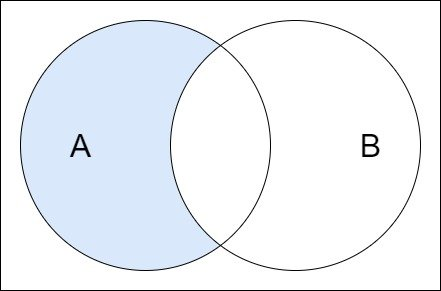### Complement

The complement of a set A is a set of all elements that do not belong to A. It's denoted in multiple ways, such as A', Ā, AC etc. Visually: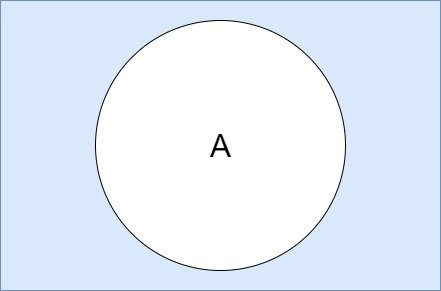### Symmetric Difference

The symmetric difference of sets A and B, denoted by A ⨁ B, is a set which contains elements that belong to A or B but not both. Visually: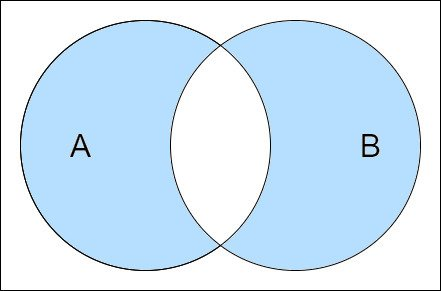## Properties of Set Operations

The set operations mentioned satisfy many properties or Laws of Algebra, which are quite useful and listed in the table below.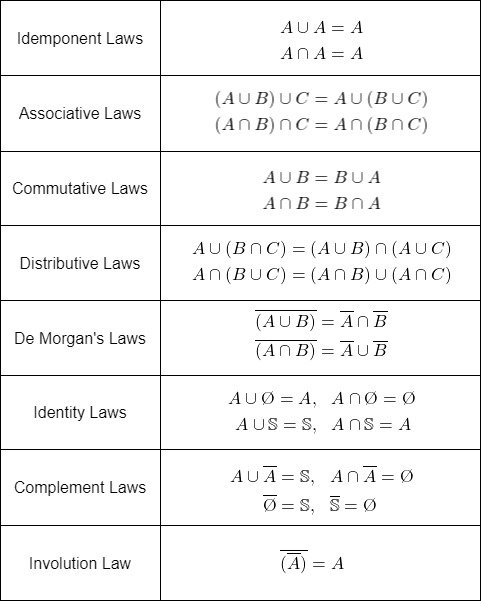In this table the calligraphic S denotes the universal set of all elements. Other commonly used symbols are V and U. Of course, this doesn't define what exactly "everything" is. If the only concern are natural numbers, then using N would make it easier to comprehend the valid numbers. Similarly, if the numbers of the sets are only integers, the integer set Z would be used instead.

## Exercise

Consider the following Venn diagram.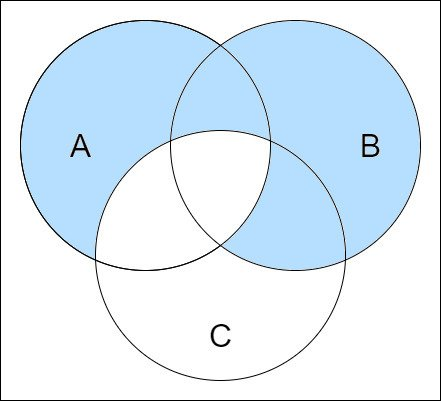Write an equation for the marked region.

## RESOURCES:

### Images

Block diagrams and other visualizations were made using draw.io.

## Previous articles of the series

• Introduction → Discrete Mathematics, Why Discrete Math, Series Outline
• Sets → Set Theory, Sets (Representation, Common Notations, Cardinality, Types)

## Final words | Next up

And this is actually it for today's post!

Next time we will continue on with more on Sets...

See ya!Keep on drifting!

Posted with STEMGeeks

Sort:

Thanks for sharing, this is kind of complicated for me :0

!1UP#### You have received a 1UP from @ivarbjorn!

The following @oneup-cartel family members will soon upvote your post:
`@stem-curator`
And they will bring !PIZZA 🍕